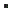AnUnt::GetBalanceEx (Interface: AnUnt)Gets the balance of this Analytical Unit Account (extended).DOUBLE GetBalanceEx (    BSTR bsAccount,    BSTR bsUnit,    BSTR bsKind,    DATE dStartDate,    DATE dEndDate)

Parameters
bsAccount
[in] The general account of which you want to know the balance.
bsUnit
[in] The analytical unit of which you want to know the balance.
bsKind
[in] The analytical kind of which you want to know the balance.
dStartDate
[in] The start date of the period for which you want to know the balance.
dEndDate
[in] The end date of the period for which you want to know the balance.

Return value
A DOUBLE containing the balance of the given analytical unit.

RemarksIf bsAccount is left blank, then the balance for all general accounts is returned.If bsUnit is left blank, then the balance for all analytical units is returned.If bsKind is left blank, then the balance for all analytical kinds is returned. In this case, the wildcard '*' can be used in the parameter bsUnit to take only those analytical units into account that begin with the characters before the wildcard.If dStartDate and dEndDate are left blank, then the balance for the complete financial year is returned.If the given dates are not included in the financial year, a balance of 0 is returned.The amount that is not distributed analytically is excluded from the returned balance.CreateAnUntAnUnt.GetBalanceAnAcc.GetBalanceC++ COleDateTime odtStartDate, odtEndDate; double lfBalance; // Get the balance of the analytical unit with code = 'UNIT' limited to general account '600', all analytical kinds and the month of January odtStartDate.SetDate (2022, 1, 1); odtEndDate.SetDate (2022, 1, 31); lfBalance = pAnUnt->GetBalanceEx ("600", "UNIT", "", odtStartDate, odtEndDate); // Get the balance of the analytical unit with code = 'UNIT' limited to all general accounts, analytical kind 'KIND' and the complete financial year lfBalance = pAnUnt->GetBalanceEx ("", "UNIT", "KIND", COleDateTime (0, 0, 0, 0, 0, 0).m_dt, COleDateTime (0, 0, 0, 0, 0, 0).m_dt); // Get the balance of all analytical units, limited to all general accounts, analytical kind 'KIND' en the first quarter of the financial year odtStartDate.SetDate (2022, 1, 1); odtEndDate.SetDate (2022, 3, 31); lfBalance = pAnUnt->GetBalanceEx ("", "", "KIND", odtStartDate, odtEndDate); // Get the balance of all analytical units, limited to all general accounts, all analytical kinds and the complete financial year lfBalance = pAnUnt->GetBalanceEx ("", "", "", COleDateTime (0, 0, 0, 0, 0, 0).m_dt, COleDateTime (0, 0, 0, 0, 0, 0).m_dt);C# DateTime dtStartDate, dtEndDate; double lfBalance; // Get the balance of the analytical unit with code = 'UNIT' limited to general account '600', all analytical kinds and the month of January dtStartDate = new DateTime (2022, 1, 1); dtEndDate = new DateTime (2022, 1, 31); lfBalance = oAnUnt.GetBalanceEx ("600", "UNIT", "", dtStartDate, dtEndDate); // Get the balance of the analytical unit with code = 'UNIT' limited to all general accounts, analytical kind 'KIND' and the complete financial year lfBalance = oAnUnt.GetBalanceEx ("", "UNIT", "KIND", new DateTime (), new DateTime ()); // Get the balance of all analytical units, limited to all general accounts, analytical kind 'KIND' en the first quarter of the financial year dtStartDate = new DateTime (2022, 1, 1); dtEndDate = new DateTime (2022, 3, 31); lfBalance = oAnUnt.GetBalanceEx ("", "", "KIND", dtStartDate, dtEndDate); // Get the balance of all analytical units, limited to all general accounts, all analytical kinds and the complete financial year lfBalance = oAnUnt.GetBalanceEx ("", "", "", new DateTime (), new DateTime ());VBS Dim lfBalance Dim dtStartDate, dtEndDate ' Get the balance of all analytical units, limited to all general accounts, all analytical kinds and the complete financial year lfBalance = oAnUnt.GetBalanceEx("", "", "", dtStartDate, dtEndDate) ' Get the balance of the analytical unit with code = 'UNIT' limited to all general accounts, analytical kind 'KIND' and the complete financial year lfBalance = oAnUnt.GetBalanceEx("", "UNIT", "KIND", dtStartDate, dtEndDate) ' Get the balance of the analytical unit with code = 'UNIT' limited to general account '600', all analytical kinds and the month of January dtStartDate = #1/1/2022# dtEndDate = #1/31/2022# lfBalance = oAnUnt.GetBalanceEx("600", "UNIT", "", dtStartDate, dtEndDate) ' Get the balance of all analytical units, limited to all general accounts, analytical kind 'KIND' en the first quarter of the financial year dtStartDate = #1/1/2022# dtEndDate = #3/31/2022# lfBalance = oAnUnt.GetBalanceEx("", "", "KIND", dtStartDate, dtEndDate)VB.NET Dim lfBalance As Double Dim dtStartDate, dtEndDate As Date ' Get the balance of all analytical units, limited to all general accounts, all analytical kinds and the complete financial year lfBalance = oAnUnt.GetBalanceEx("", "", "", dtStartDate, dtEndDate) ' Get the balance of the analytical unit with code = 'UNIT' limited to all general accounts, analytical kind 'KIND' and the complete financial year lfBalance = oAnUnt.GetBalanceEx("", "UNIT", "KIND", dtStartDate, dtEndDate) ' Get the balance of the analytical unit with code = 'UNIT' limited to general account '600', all analytical kinds and the month of January dtStartDate = #1/1/2022# dtEndDate = #1/31/2022# lfBalance = oAnUnt.GetBalanceEx("600", "UNIT", "", dtStartDate, dtEndDate) ' Get the balance of all analytical units, limited to all general accounts, analytical kind 'KIND' en the first quarter of the financial year dtStartDate = #1/1/2022# dtEndDate = #3/31/2022# lfBalance = oAnUnt.GetBalanceEx("", "", "KIND", dtStartDate, dtEndDate)